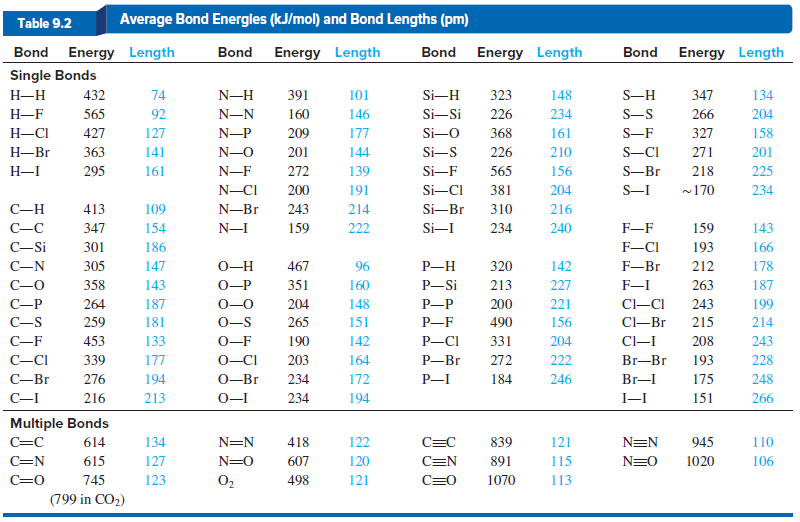# Problem: Ethanol (CH3CH2OH) is being used as a gasoline additive or alternative in many parts of the world.(a) Use bond energies to find ΔH°rxn for the combustion of gaseous ethanol. (Assume H 2O forms as a gas.)(b) In its standard state at 25°C, ethanol is a liquid. Its vaporization requires 40.5 kJ/mol. Correct the value from part (a) to find the enthalpy of reaction for the combustion of liquid ethanol.(c) How does the value from part (b) compare with the value you calculate from standard enthalpies of formation (Appendix B)?

###### Problem Details

Ethanol (CH3CH2OH) is being used as a gasoline additive or alternative in many parts of the world.
(a) Use bond energies to find ΔH°rxn for the combustion of gaseous ethanol. (Assume H 2O forms as a gas.)
(b) In its standard state at 25°C, ethanol is a liquid. Its vaporization requires 40.5 kJ/mol. Correct the value from part (a) to find the enthalpy of reaction for the combustion of liquid ethanol.
(c) How does the value from part (b) compare with the value you calculate from standard enthalpies of formation (Appendix B)?